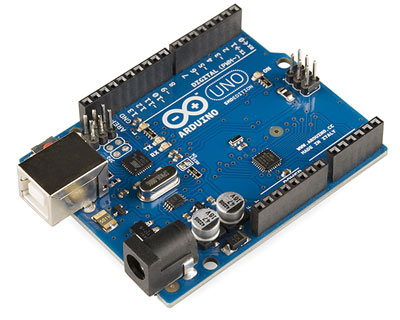# Arduino Based Digital Thermometer

Thermometers are useful apparatus being used since long time for temperature measurement. In this project we have made an Arduino based digital thermometer to display the current ambient temperature and temperature changes on a LCD unit in real time .It can be deployed in houses, offices, industries etc. to measure the temperature. This project is based on Arduino which communicates here with LM35 temperature sensor and a 16×2 display unit. We can divide this arduino based thermometer into three sections – one senses the temperature by using temperature sensor LM 35, second section converts the temperature value into a suitable numbers in Celsius scale which is done by Arduino, and last part of system displays temperature on LCD. The same is demonstrated in below block diagram.Arduino Uno is used here to control the whole process. An LM35 temperature sensor is used for sensing environment temperature which gives 1 degree temperature on every 10mV change at its output pin. You can easily check it with voltmeter by connecting Vcc at pin 1 and Ground at pin 3 and output voltage at pin 2 of LM35 sensor. For an example if the output voltage of LM35 sensor is 250m volt, that means the temperature is around 25 degree Celsius.

Arduino reads output voltage of temperature sensor by using Analog pin A0 and performs the calculation to convert this Analog value to a digital value of current temperature. After calculations arduino sends these calculations or temperature to 16×2 LCD unit by using appropriate commands of LCD.

### Circuit Components

Arduino

In this project we have used a microcontroller to control whole the process of system that is ARDUINO board. Actually arduino is not a mere controller as it has an operating system or boot-loader which runs on AVR controllers. Arduino is an open source hardware platform and very useful for project development purpose. There are many types of arduino boards like Arduino UNO, arduino mega, arduino pro mini, Lilypad etc. are available in the market or you can also build one by yourself.LM35 Temperature Sensor

LM35 is a 3 pin temperature sensor which gives 1 degree Celsius on every 10mVolt change. This sensor can sense up to 150 degree Celsius temperature. 1 number pin of lm35 sensor is Vcc, second is output and third one is Ground.

Read More:  Arduino Based Digital Thermometer

#### This Post / Project can also be found using search terms:

• arduino based digital thermometer
• arduino thermometer